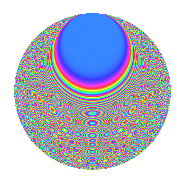# Properties

 Label 1350.2.lLevel 1350 Weight 2 Character orbit l Rep. character $$\chi_{1350}(151,\cdot)$$ Character field $$\Q(\zeta_{9})$$ Dimension 342 Sturm bound 540

# Related objects

## Defining parameters

 Level: $$N$$ = $$1350 = 2 \cdot 3^{3} \cdot 5^{2}$$ Weight: $$k$$ = $$2$$ Character orbit: $$[\chi]$$ = 1350.l (of order $$9$$ and degree $$6$$) Character conductor: $$\operatorname{cond}(\chi)$$ = $$27$$ Character field: $$\Q(\zeta_{9})$$ Sturm bound: $$540$$

## Dimensions

The following table gives the dimensions of various subspaces of $$M_{2}(1350, [\chi])$$.

Total New Old
Modular forms 1692 342 1350
Cusp forms 1548 342 1206
Eisenstein series 144 0 144

## Trace form

 $$342q + 6q^{6} - 3q^{8} + 12q^{9} + O(q^{10})$$ $$342q + 6q^{6} - 3q^{8} + 12q^{9} + 9q^{11} - 3q^{12} + 6q^{14} - 12q^{17} + 6q^{18} - 48q^{21} - 9q^{22} + 48q^{23} - 36q^{26} + 39q^{27} + 42q^{29} + 18q^{31} + 39q^{33} - 9q^{34} - 6q^{36} + 24q^{38} + 42q^{39} + 9q^{41} + 24q^{42} - 9q^{43} + 6q^{44} + 72q^{47} + 6q^{48} + 18q^{49} + 84q^{51} + 96q^{53} + 6q^{56} + 27q^{57} + 54q^{59} + 18q^{61} - 24q^{62} + 78q^{63} - 171q^{64} - 45q^{67} + 24q^{68} - 102q^{69} + 24q^{71} - 24q^{72} - 18q^{73} - 66q^{74} + 18q^{76} - 102q^{77} + 60q^{78} - 72q^{79} + 96q^{81} + 60q^{83} + 9q^{86} - 96q^{87} + 18q^{88} + 129q^{89} - 18q^{91} - 42q^{92} + 42q^{93} - 36q^{94} + 6q^{96} - 45q^{97} - 15q^{98} + 72q^{99} + O(q^{100})$$

## Decomposition of $$S_{2}^{\mathrm{new}}(1350, [\chi])$$ into newform subspaces

The newforms in this space have not yet been added to the LMFDB.

## Decomposition of $$S_{2}^{\mathrm{old}}(1350, [\chi])$$ into lower level spaces

$$S_{2}^{\mathrm{old}}(1350, [\chi]) \cong$$ $$S_{2}^{\mathrm{new}}(27, [\chi])$$$$^{\oplus 6}$$$$\oplus$$$$S_{2}^{\mathrm{new}}(54, [\chi])$$$$^{\oplus 3}$$$$\oplus$$$$S_{2}^{\mathrm{new}}(135, [\chi])$$$$^{\oplus 4}$$$$\oplus$$$$S_{2}^{\mathrm{new}}(270, [\chi])$$$$^{\oplus 2}$$$$\oplus$$$$S_{2}^{\mathrm{new}}(675, [\chi])$$$$^{\oplus 2}$$

## Hecke Characteristic Polynomials

There are no characteristic polynomials of Hecke operators in the database